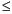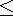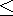Functions and CALL Routines

# CALL RANBIN Routine

Returns a random variate from a binomial distribution.
 Category: Random Number

## Syntax

 CALL RANBIN(seed,n,p,x);

### Arguments

seed

is the seed value. A new value for seed is returned each time CALL RANBIN is executed.

 Range: seed < 231 - 1 Note: If seed0, the time of day is used to initialize the seed stream. See: Seed Values and Comparison of Seed Values in Random-Number Functions and CALL Routines for more information about seed values
n

is an integer number of independent Bernoulli trials.

 Range: n > 0
p

is a numeric probability of success parameter.

 Range: 0
x

is a numeric SAS variable. A new value for the random variate x is returned each time CALL RANBIN is executed.

The CALL RANBIN routine updates seed and returns a variate x that is generated from a binomial distribution with mean np and variance np(1-p). If n50, np5, or n(1-p)5, SAS uses an inverse transform method applied to a RANUNI uniform variate. If n>50, np>5, and n(1-p)>5, SAS uses the normal approximation to the binomial distribution. In that case, the Box-Muller transformation of RANUNI uniform variates is used.

By adjusting the seeds, you can force streams of variates to agree or disagree for some or all of the observations in the same, or in subsequent, DATA steps.

For a discussion and example of an effective use of the random number CALL routines, see Starting, Stopping, and Restarting a Stream.

The CALL RANBIN routine gives greater control of the seed and random number streams than does the RANBIN function.

The following example uses the CALL RANBIN routine:

```options pageno=1 nodate ls=80 ps=64;

data u1 (keep = x);
seed = 104;
do i = 1 to 5;
call ranbin(seed, 2000, 0.2 ,x);
output;
end;
call symputx('seed', seed);
run;

data u2 (keep = x);
seed = &seed;
do i = 1 to 5;
call ranbin(seed, 2000, 0.2 ,x);
output;
end;
run;

data all;
set u1 u2;
z = ranbin(104, 2000, 0.2);
run;

proc print label;
label x = 'Separate Streams' z = 'Single Stream';
run;```

```                                 The SAS System                                1

Separate    Single
Obs     Streams    Stream

1       423        423
2       418        418
3       403        403
4       394        394
5       429        429
6       369        369
7       413        413
8       417        417
9       400        400
10       383        383
```

 Functions:Previous Page | Next Page | Top of Page